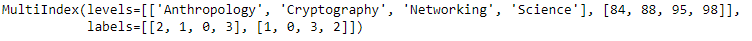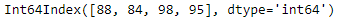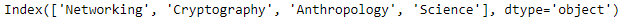Related Articles
Python | Pandas MultiIndex.droplevel()
• Last Updated : 24 Dec, 2018

Python is a great language for doing data analysis, primarily because of the fantastic ecosystem of data-centric python packages. Pandas is one of those packages and makes importing and analyzing data much easier.

Pandas` MultiIndex.droplevel()` function return Index with requested level removed. If MultiIndex has only 2 levels, the result will be of Index type not MultiIndex..

Syntax: MultiIndex.droplevel(level=0)

Parameters :
level : int/level name or list thereof

Returns : index : Index or MultiIndex

Example #1: Use `MultiIndex.droplevel()` function to drop the 0th level of the MultiIndex.

 `# importing pandas as pd ` `import` `pandas as pd ` ` `  `# Create the MultiIndex ` `midx ``=` `pd.MultiIndex.from_arrays([[``'Networking'``, ``'Cryptography'``,  ` `                                     ``'Anthropology'``, ``'Science'``],  ` `                                             ``[``88``, ``84``, ``98``, ``95``]]) ` ` `  `# Print the MultiIndex ` `print``(midx) `

Output :Now let’s drop the 0th level of the MultiIndex.

 `# drop the 0th level. ` `midx.droplevel(level ``=` `0``) `

Output :As we can see in the output, the function has dropped the 0th level and returned an Index object.

Example #2: Use `MultiIndex.droplevel()` function to drop the 1st level of the MultiIndex.

 `# importing pandas as pd ` `import` `pandas as pd ` ` `  `# Create the MultiIndex ` `midx ``=` `pd.MultiIndex.from_arrays([[``'Networking'``, ``'Cryptography'``, ` `                                     ``'Anthropology'``, ``'Science'``], ` `                                              ``[``88``, ``84``, ``98``, ``95``]]) ` ` `  `# Print the MultiIndex ` `print``(midx) `

Output :Now let’s drop the 1st level of the MultiIndex.

 `# drop the 1st level. ` `midx.droplevel(level ``=` `1``) `

Output :As we can see in the output, the function has dropped the 1st level and returned an Index object.

Attention geek! Strengthen your foundations with the Python Programming Foundation Course and learn the basics.

To begin with, your interview preparations Enhance your Data Structures concepts with the Python DS Course.

My Personal Notes arrow_drop_up
Recommended Articles
Page :